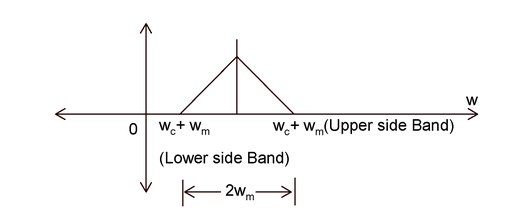# LSB (Lower Side Band) is the band of frequency

## LSB (Lower Side Band) is the band of frequency

a.) Below the carrier frequency
b.) Includes the carrier frequency
c.) That lies in AM spectrum
d.) Both a) and c) are correct

Correct Answer: d) Both a) and c) are correct

Explanation:LSB (Lower Side Band) is the band of frequency below the carrier frequency that lies in AM spectrum. It is a part of the shifted spectrum obtained after amplitude modulation. It is denoted by ωc- ωm where ωc is the carrier frequency and ωm is the modulating frequency.